#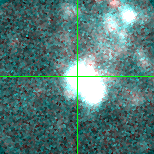M33-5 (J013316.57+305250.2)

## Target Notes### Classification: -

Khan et al. 2012. J013316.59+305249.9 of comparable brightness within one arc second. Four other stars one magnitude or fainter within 2 arc seconds.

## Plots of Brightness and Color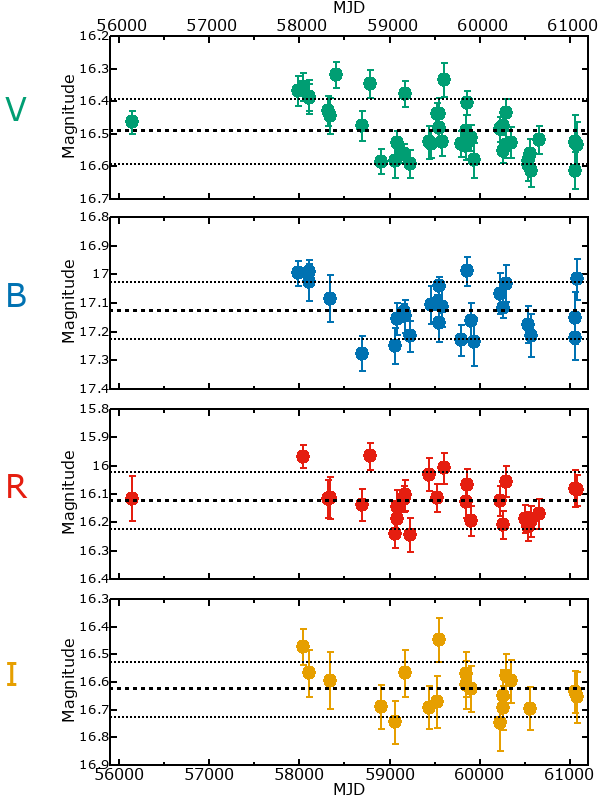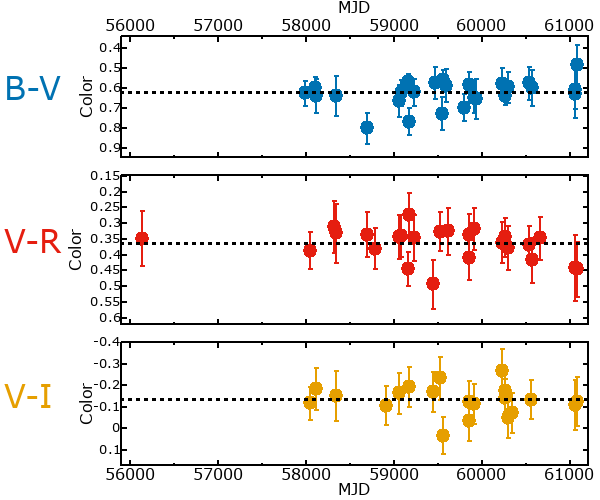## Variability

It is likely that this star is variable.

### Correlation factors between bands

If the brightness variation is true variability then there should be a correlation between brightness changes recorded in different filter bands. The following table are the R-squared linear correlation factors calculated for the pairs of bands. The number in parentheses next to the R-squared value is the number of elements used to calculate the correlation factor. The number in square brackets next to the band is the range of magnitudes measured.

V [0.62 mag]B [0.29 mag]R [0.30 mag]
B [0.62 mag]0.03 (10)
R [0.30 mag]0.78 (9)0.42 (7)
I [0.55 mag]0.82 (6)0.98 (4)0.39 (5)

### Magnitude-Magnitude Plots

The plots below are provided to check the values in the table above and also to show any non-linear correlations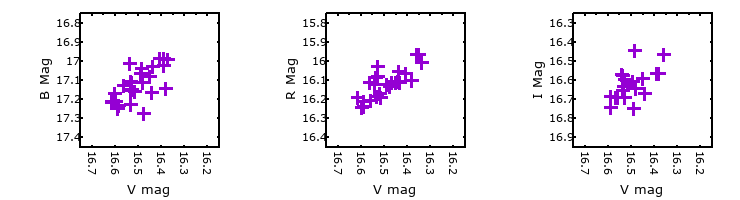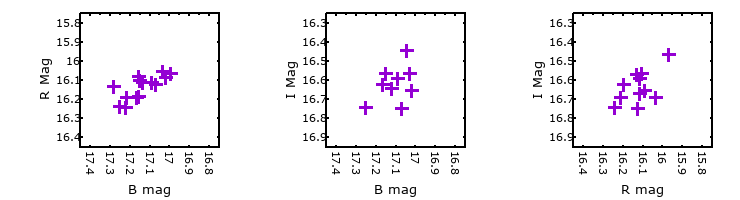### I & R Statistics

Welch & Stetson (1993, AJ, 105, 1813) present a method for varible star detection in CCD images from automated surveys. Data from two bands are compared and two statistics are calculated: R and I.

The I-statistic has an expectation value of zero for stars that are NOT variable. A larger non-zero I-value indicates a higher likelihood that the star is variable. The R statistic indicates how the color of the star changes as the variable grows brighter with R = 1.0 being no change in color.

R and I values have only been calculated if there are more than four (4) matched measurements of brightness in each band.
V
(I|R)
B
(I|R)
R
(I|R)
B
(I|R)
0.72 | 2.11
R
(I|R)
2.66 | 0.81-3.09 | 0.08
I
(I|R)
1.12 | 0.97--- | --- 2.57 | 0.61